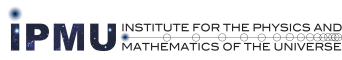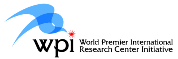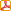### IPMU Seminar

 Date: October 2, 2008, 15:30 - 17:00 Place: Seminar Room at IPMU Prefab. B, Kashiwa Campus of the University of Tokyo Speaker: Sven Bjarke Gudnason (Univ. of Pisa) Title: SO and USp K\"ahler and HyperK\"ahler Quotients and LumpsAbstract: The properties of supersymmetric gauge theories in the Higgs phase at low energies can appropriately be studied by means of a non-linear sigma model, which has the target space being K\"ahler for N=1 supersymmetric models and hyper-K\"ahler for N=2 models. By construction of the K\"ahler and hyper-K\"ahler quotients for the gauge theories with SO and USp gauge symmetries, we obtain the explicit metrics and their K\"ahler potentials of their corresponding manifolds, which are equivalent to the moduli space of vacua of the original gauge theories. Furthermore, we develop an expansion formula to make use of the obtained metrics from which we also calculate the curvatures of the manifolds. The last scope is to study the lumps in the non-linear sigma models and their effective descriptions. In this connection we introduce the moduli matrix formalism and make a connection with the moduli space of vortices from the moduli space of lumps.